Electricity Flashcards Preview

Physics > Electricity > Flashcards

Flashcards in Electricity Deck (108):
1

2

3

4

5

6

7

8

9

A1 = 0.18 Ampswhat is the Amps of A2 and A3why?10

A1 = 0.34 Ampswhat is the Amps of A2 and A3? why?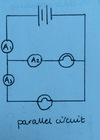11

12

13

V1 = 2.73 voltsV2 = 1.00 voltswhat is V3 ?why?14

what happens to V2 when you remove one of the bulbs?15

what happens to V2 when you remove a cell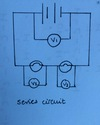16

V1 = 1.65 Voltswhat are V2 and V3 ?why?17

complete the data for all of the An knowing:A3 = 2.5 AmpsA5 = 1.5 AmpsA6 = 2.0 Amps18

19

20

21

22

23

24

25

26

27

28

29

30

31

given that V1 = 3.0 V what is V2, V3 and V4?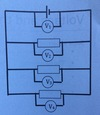32

given that V2 = 1.5V, V3 = 1.5V and V4 = 1.5V, what is V1?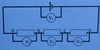33

consider a coulomb of charge in the cellit has 4.5J of electrical energy when it leaves the cell and moves around to position A in the circuitposition A has 4.5J (Coulomb energy)how much Coulomb energy does B, C and D have?V1 = 4.5V2, V3, V4 = 1.534

35

36

37

38

39

what is the symbol for a resistor?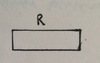40

what is the symbol for a variable resistor?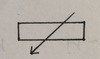41

42

43

on a graph, does a higher resistance has a bigger or smaller gradient?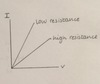44

45

46

47

48

49

explain why we get his curve on the I-V graph for a filament lamp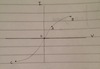the resistance for B gets larger as the lamp gets hotter (thermal energy  - energy disipated from circuit) as it gets brighterthe positive ions (which metals are made up of) vibrate morethe lower the gradient the higher the resistance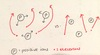50

the terminals of the power supply on a circuit for a filament lamp are reversed which produces data O--> Cdoes the filament bulb's characteristics change due to the change in current dicrection?51

52

53

54

what is the symbol for a L.D.R?55

how do you set up an experiment to record the resistance of a L.D.R.?

shine a light on the L.D.R. and record the resistanve using the ohm-meterplace tracing paper between the lamp and the L.D.R. to reduce the light intensity ad record the resistance of the L.D.R.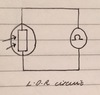56

57

what is the symbol for a thermistor?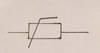58

59

resistance and temperature are ... what does this look like on a graph?

inversely proptional60

61

what is the symbol for a diode?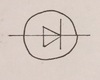62

63

64

what does the circuit look like to test the  current and voltage across a diode?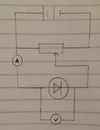65

what does an I-V graph look like in an experiment including diodeexplain

in reverse bias the current in very low therefore the resistance is very highin foward bias the current is high therefore the resistance is lowcurrent suddenly increase at 0.7 volts (resistance suddenly decreases)66

a student wants to vary the pd across a 2Ω resistor to record how the current varies with pdhow does the student vary the pd usingjust the equiptment in the circuit?67

68

given that the voltmeter reads 4.5V, what is the pd across the variable resistor when he cells is 6V?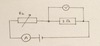69

given that the pd across the variable reistor is 1.5V and the current is 2.25 Amps, what is the resistance (Rh) of the variable resistor?70

the student wants only L3 to workwhich switches should she close?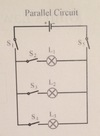71

the same 6V battery is used to power 3 identical light bulbs in a parallel ciruitwhat is the pd across L372

73

74

75

explain why a filament lamp's I-V characteristics are as shown

the filament lamp does not obey Ohm's law as the current and voltage are not directly proportionalwe get the curve shape because the resistance for B gets larger as the lamp gets hotter (thermaln energy) as it gets brighterthe graph shows an inverse relationship as the gradient gets lower as the resistance gets higheras current increases, temperature increasesthe terminals of the power supply are reversed which produces data 0-Cthe filament lamp has rotational symmetry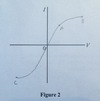76

77

78

79

80

81

82

83

84

85

86

87

88

89

draw an I-V graph for a resistor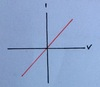90

draw an I-V graph for a filament lamp91

draw an I-V graph for a diode92

93

94

Briefly: metal filament lamp on an I-V graph (explain resistance)

as the temperature of the meta filament increases, the resistance increases, hence the curve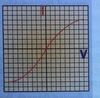95

Briefly: a wire on an I-V graph (expalin resistance)

the current through a wire (at constant temperature) is proportional to voltage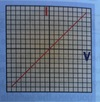96

Briefly: different resistors on an I-V graph (expalin resistance)

the current through a resistor (at constat temperature) is proportional to voltagedifferent resistors have different resistances, hence the different slopes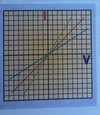97

Briefly: a diode on an I-V graph (expalin resistance)

current will only flow through a diode in one direction, as shown98

99

100

101

102

103

104

105

106

107

What happens when an electrical charge goes through a change in voltage?

when an electrical charge goes through a change in voltage, energy is transferredenergy is supplied to the charge at the power source to 'raise' it through a voltagethe charge gives up this energy when it 'falls' through any voltage drop in components elsewhere in the circuitthe bigger the change in voltage, the more energy is transferred for a given amount of charge passing through a circuit108